# Rocking Response of Seismically-Isolated Rigid Blocks Under Simple Acceleration Pulses and Earthquake Excitations

Panayiotis C. Roussis*, Spyroulla Odysseos
Department of Civil and Environmental Engineering, University of Cyprus, Nicosia, CY-1678, Cyprus

#### Article Metrics

0
##### Total Statistics:

Full-Text HTML Views: 2006
Abstract HTML Views: 1407
##### Unique Statistics:

Full-Text HTML Views: 1036
Abstract HTML Views: 814open-access license: This is an open access article distributed under the terms of the Creative Commons Attribution 4.0 International Public License (CC-BY 4.0), a copy of which is available at: https://creativecommons.org/licenses/by/4.0/legalcode. This license permits unrestricted use, distribution, and reproduction in any medium, provided the original author and source are credited.

* Address correspondence to this author at the Department of Civil & Environmental Engineering, University of Cyprus, Nicosia, 1 Panepistimiou Avenue, 2109 Nicosia, Cyprus; Tel: +357 22892210, E-mail: roussis@ucy.ac.cy

## Abstract

### Background:

Although the dynamic response of rigid block-like structures standing free on a rigid foundation has been extensively studied to date, only a limited number of studies have focused on the dynamics of such systems when seismically isolated.

### Objective:

This paper presents a comprehensive investigation on the dynamic response of base-isolated rigid blocks subjected to pulse-type base excitation, with the aim of identifying potential trends in the response and stability of the system.

### Method:

The model adopted in this study consists of a rectangular-prismatic rigid block standing free on a seismically-isolated base, which, on the assumption of sufficiently-large friction, can be set into rocking on top of the moving base under dynamic excitation. The study examines in depth the motion of the block/base system with a large-displacement formulation that combines the nonlinear equations of motion with a rigorous model governing impact. Two isolation-system models are utilized in the analysis, a linear viscoelastic model and a bilinear hysteretic model.

### Results:

An extensive numerical investigation was performed to calculate the rocking response of the block under simple acceleration pulses and recorded pulse-type earthquake motions of various amplitudes and frequency content. Response-regime spectra for non-isolated and isolated blocks of varying geometric characteristics have been constructed to evaluate the system performance with respect to the rocking initiation and overturning of the block.

### Conclusion:

The study showed that, regardless of block size and excitation period, seismic isolation increases the acceleration required to initiate rocking, a benefit that increases as the isolation period increases. In regard to the stability of the rocking block, the use of isolation yields a better system performance for smaller-sized blocks both for short- and mid-period excitations, provided that the isolation system is suitably designed. On the contrary, for long-period pulses, the use of isolation is practically not beneficial in improving the stability of the rocking block, irrespective of its size.

Keywords: Seismic isolation, Rigid block, Rocking, Impact, Overturning, Pulse-type base excitation.

## 1. INTRODUCTION

A large number of research papers in the literature have been devoted to the study of the dynamic behavior of rigid block-like structures to base excitation. Housner's landmark study  constitutes perhaps the first systematic work that provided basic understanding on the rocking response of a rigid block and motivated further scientific interest e.g. [2-12]. Considering a slender rigid block resting upon a rigid foundation, based on the assumption of perfectly-inelastic impact and sufficient friction to prevent sliding, Housner investigated the free- and forced-vibration rocking response to a rectangular pulse, a half-sine pulse, and an earthquake-type excitation. More recently, motivated primarily by the need to mitigate the seismic risk of objects of cultural heritage, a rather limited number of studies on the rocking response of a rigid block with its base seismically isolated have been pursued e.g. [13-17].

This paper presents a comprehensive mathematical formulation for the nonlinear rocking response of seismically-isolated free-standing rigid blocks to base excitation. The study examines in depth the system response based on a large-displacement formulation that combines the nonlinear equations of motion together with a rigorous model governing impact. The mathematical treatment of the problem is broad in scope in that it is neither restricted to small rotations nor slender blocks. Despite the apparent geometric simplicity of the problem, the mathematical description of the system dynamics is profoundly complex, mainly due to the inherent nonlinear nature of the impact phenomenon and the potential transition from one oscillation pattern to another, each one governed by different equations of motion. A rigorous formulation of the impact problem is presented in this paper based on the study originally published by Roussis et al. . Derived from first principles, the model assumes point-impact, perfectly-inelastic impact (i.e. zero coefficient of restitution), and Coulomb-type friction sufficiently large so that sliding of the block during impact is prevented. The model elucidates the inherent base-block dynamic interaction, a fundamental response feature that distinguishes the problem at hand from the classic Housner-type problem of a rocking block impacting a rigid foundation with infinite mass. To the authors’ best knowledge, this model is the first to treat correctly from a theoretical point of view this important aspect of the system dynamic response. Based on the developed model, a computer program was developed in Matlab to calculate the system response to simple acceleration pulses and pulse-type earthquake motions of various amplitudes and frequency content. An extensive numerical investigation was carried out for different geometric characteristics of the block and isolation-system parameters, aiming to identify potential trends in the rocking response and stability of the system.

## 2. ANALYTICAL FORMULATION

### 2.1. Model Description

The adopted model consists of a rectangular-prismatic rigid block of mass m and centroid mass-moment of inertia IC , standing free on a seismically-isolated rigid base of mass mb (Fig. (1a)). The block of height H = 2h and width B = 2b is assumed to rotate about the corners O and O'. A measure of the size of the block is given by the half-diagonal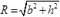of the rectangle, while a measure of its slenderness is given by the height-to-width ratio λ = h/b or equivalently by the angle α = tan-1 (b/h). A measure of the dynamic characteristics of the block, albeit in an approximate sense since the natural frequency is amplitude-dependent, is given by the size parameter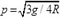. Two isolation-system models are utilized in the analysis: (a) a linear viscoelastic model, defined by parameters kb and cb (presented in Section 2.2.1), and (b) a bilinear hysteretic model (typified by the friction-pendulum type), defined by parameters µb and Rb (presented in Section 2.2.2).

Assuming no sliding of the block against the rigid base, when subjected to ground excitation the system can exhibit two possible oscillation patterns: (a) full-contact, during which the block/base system oscillates as one unit horizontally with displacement u(t)— 1DOF response (Fig. (1b)), and (b) rocking, during which the rigid block pivots on its edges with rotation angle θ(t) as the supporting base translates horizontally with u(t)— 2DOF response (Fig. (1c)). The rotation angle of the block is denoted by θ(t), positive in the clockwise sense, and the horizontal displacement of the base with respect to the foundation is denoted by u(t).

### 2.2. Equations of Motion

When subjected to horizontal ground acceleration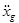, the supporting base will oscillate in the horizontal direction with a displacement u(t) relative to the foundation. The rigid block will be set into rocking on top of the moving base when the overturning moment due to external loads exceeds the resisting moment due to gravity, yielding the following condition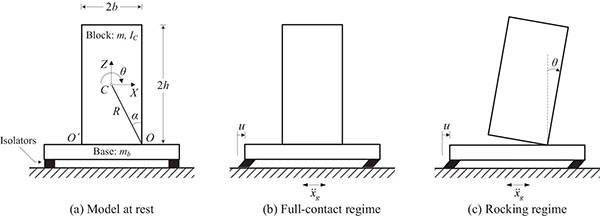Fig. (1). Model at rest and oscillation patterns considered.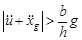(1)

If the total acceleration of the base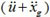is positive, then rocking of the block takes place about corner O', or else if it is negative rocking takes place about corner O.

The governing equations for each regime of motion are derived next through a large-displacement formulation (not restricted to slender blocks) by means of the Lagrange method, for two seismic-isolation models. The problem description will be presented in terms of the geometric parameters of the block (b, h), the inertia parameters of the system (m, mb), the constitutive parameters of the isolation system (kb, cb or µb, Rb), and the horizontal component of ground acceleration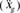.

#### 2.2.1. Linear Model for Isolation System

Consider first the block isolated with a linear isolation system composed of a linear spring with stiffness kb and a linear viscous damper with coefficient cb, by interposing a rigid base of mass mb. The behavior of such a linear-viscoelastic model in terms of the lateral force developed in the isolation system is described by: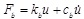(2)

The equation governing the response in the full-contact regime, in which the block remains in full contact with the horizontally moving base, is given by: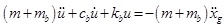(3)

Equation (3) is the classical second-order differential equation governing the response of a single-degree-of-freedom system of mass (m+mb) to ground excitation. The (isolation) system period Tb and damping ratio ξb are given by the following expressions, respectively: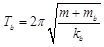(4)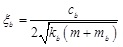(5)

In the rocking regime, the system possesses two degrees of freedom. Using as generalized coordinates q1 ≡ u, the horizontal translation of the base relative to the ground, and q2 ≡ θ, the rotation angle of the block about a base corner, Lagrange’s equations take the form: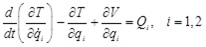(6)

in which T denotes the kinetic energy of the system, V the potential energy of the system, and Qi the generalized non-conservative forces.

For the case when the block rotates about corner O (θ > 0), the kinetic energy of the system is obtained as: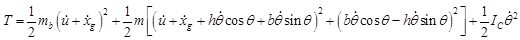(7)

in which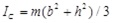for a rectangular block.

The first term in Eq. (7) is associated with the horizontal translation of the base, and the second and third terms are associated with the general planar motion of the block (composed of a pure-translation component and a pure-rotation component, both with reference to the mass center of the block).

The potential energy of the system is obtained as: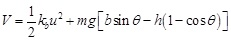(8)

The generalized forces Qi are derived through the virtual work of the non-conservative forces as: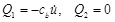(9)

Substituting the appropriate derivatives of the kinetic and potential energy of the system (Eqs. (7) and (8)), together with the expressions for the non-conservative forces (Eq. (9)), into Lagrange’s equations (Eq. (6)) gives the equations of motion for rotation about O (θ > 0). The equations of motion for rotation about O' (θ < 0) can be derived in a similar manner. Combining the equations for rotation about O and O', leads to the following compact set of equations governing the rocking regime of the block over of the translating base: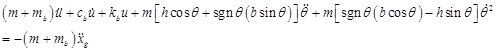(10)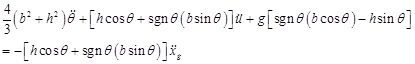(11)

where sgnθ denotes the signum function in θ.

Evidently, the mutually-coupled equations governing the rocking regime are highly nonlinear and not amenable to closed-form solution even for the simplest form of ground excitation. Note that Eqs. (10) and (11) hold only in the absence of impact (θ ≠ 0). At that instant, both corner points O and O' are in contact with the base, rendering the above formulation invalid. The impact problem is addressed separately in Section 2.3.

#### 2.2.2. Nonlinear Model for Isolation System

Consider the bilinear hysteretic model to represent the mechanical behavior of friction-pendulum-type isolation system, described by: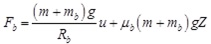(12)

where Rb is the radius of curvature and µb is the coefficient of friction of the friction-pendulum (FP) isolators; and Z is a dimensionless variable describing the rigid-plastic behavior being governed by the following differential equation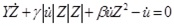(13)

in which Y is the yield displacement, and β, γ are dimensionless parameters that control the shape of the hysteresis loop, with assigned values: β = 0.1, γ = 0.9, Υ = 0.3mm .

In Eq. (12), the coefficients of u and Z represent the second slope of the bilinear model and the strength of the system, respectively.

The equation governing the response in the full-contact regime is readily obtained as: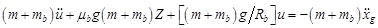(14)

In the rocking regime, for the case when the block rotates about corner O (θ > 0), the kinetic energy of the system is again given by Eq. (7), while the potential energy and the generalized forces of the system are derived respectively as: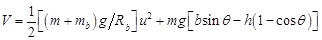(15)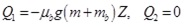(16)

Substituting the expressions for the kinetic energy, the potential energy, and the non-conservative forces of the system into Lagrange’s equations yields the governing equations of motion for rotation about O (θ > 0). Analogous procedure can be followed for deriving the governing equations of motion for rotation about O' (θ < 0). A combined set of equations governing rocking of the block about O and O' on top of the moving base (valid for θ ≠ 0) takes the form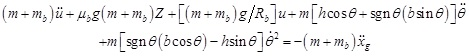(17)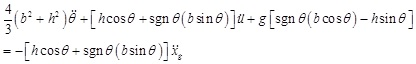(18)

where sgn θ denotes the signum function in θ.

### 2.3. Impact Model

A rigorous mathematical formulation of the impact problem is presented in this paper, based on the study originally published by Roussis et al. . To the authors’ best knowledge, this model was the first to treat correctly from the theoretical point of view this important aspect of the system dynamic response.

The dynamic response of the system is strongly affected by the occurrence of impact of the rocking block on the horizontally-moving base. In fact, impact affects the system response on many different levels. On one level, it renders the problem highly nonlinear (aside from the nonlinear nature of the equations themselves) by virtue of the discontinuity introduced in the response (i.e. the governing equations of motion cease to be valid at θ = 0). As a result, impact causes the system to switch from one oscillation pattern to another (potentially modifying the degrees of freedom), each one governed by a different set of differential equations. This in turn entails that the integration of equations of motion governing the post-impact response must account for the ensuing instantaneous change of the system velocity regime. In this regard, the dynamic response is critically influenced by impact, in that impact contributes (exclusively) to the energy dissipation in the system, manifested through the reduction of the post-impact velocities.

Therefore, the critical role of impact in the dynamics of the system necessitates a rigorous formulation of the impact problem. In this paper, a model governing impact is derived from first principles using classical impact theory. According to the principle of impulse and momentum, the duration of impact is assumed short and the impulsive forces are assumed large relative to other forces in the system. Changes in position and orientation are neglected, and changes in velocity are considered instantaneous. Moreover, this model assumes point-impact, perfectly inelastic impact (i.e. zero coefficient of restitution), impulses acting only at the impacting corner (i.e. impulses at the rotating corner are small compared to those at the impacting corner and are neglected), and sufficient friction to prevent sliding of the block during impact.

Based on the assumption of perfectly inelastic impact, the block can exhibit two possible response mechanisms following impact: (a) rocking about the impacting corner, when the block re-uplifts (no bouncing), and (b) pure translation in full-contact with the base, when the block’s rocking motion ceases after impact. The formulation of impact is divided into three phases: pre-impact, impact, and post-impact, as illustrated schematically in Fig. (2). In the following, a superscript “-” refers to a pre-impact quantity and a superscript “+” to a post-impact quantity.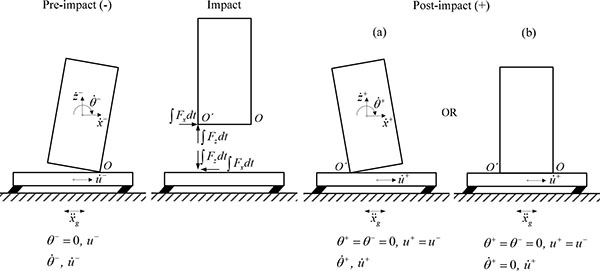Fig. (2). Impact analysis phases.

#### 2.3.1. Rocking Persists after Impact

Consider the instant at which the block hits upon the translating base from rocking about O and re-uplifts about the impacting corner, O' (Fig. (2a)). As mentioned before, impact is accompanied by an instantaneous change in velocities, with the system displacements being unchanged. Therefore, the analysis of impact is reduced to calculating the initial conditions for the post-impact motion,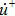and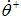, given the position and the pre-impact velocities,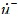and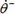.

Considering the block during impact, the application of the principle of linear impulse and momentum in the x and z direction gives the following equations: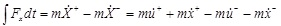(19)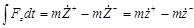(20)

in which ∫Fx dt and ∫Fz dt are the horizontal and vertical impulses (assumed to act at O');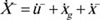,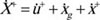, and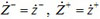are the absolute pre- and post-impact horizontal and vertical velocities of the mass center of the block, respectively, expressed in terms of their pre- and post-impact relative counterparts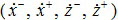, the relative-to-ground velocity of base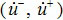, and the ground velocity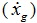.

In addition, the principle of angular impulse and momentum dictates that: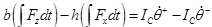(21)

In Eqs. (19) and (20), the pre- and post-impact horizontal and vertical components of the relative translational velocity of the mass center can be expressed in terms of the angular velocity of the block as: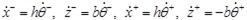(22)

Substituting Eqs. (22) into Eqs. (19)-(20) yields: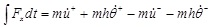(23)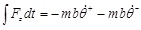(24)

Equations (21), (23), and (24) constitute a set of three equations in four unknowns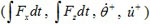. Equivalently, these equations can be combined in one equation (by eliminating the two impulses) in two unknowns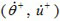yielding: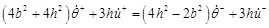(25)

in which for rectangular block the centroid mass moment of inertia was taken as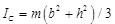.

One additional equation is therefore required to uniquely determine the post-impact velocitiesand. By considering the system in its entirety during impact, it can be stated that the horizontal impulse on the system is zero, resulting in the conservation of the system’s linear momentum in the horizontal direction. That is,: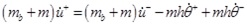(26)

Alternatively, instead of considering the conservation of linear momentum (in the horizontal direction) of the entire system, one can apply the principle of linear impulse and momentum (in the horizontal direction) of the base alone, reaching at the same result (Eq. (26)).

Combining Eqs. (25) and (26) gives the post-impact velocities of the system (i.e. the angular velocity of the block and the translational velocity of the supporting base) as: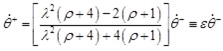(27)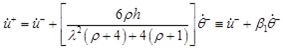(28)

in which λ = h/b is the slenderness ratio and ρ = m/mb is the mass ratio.

The coefficient of “angular restitution” ε in Eq. (27), associated with the reduction of the post-impact angular velocity of the block, is defined by: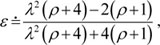(29)

and the coefficient of “linear restitution” β1 in Eq. (28), associated with the reduction of the post-impact linear velocity of the rigid base, is defined by: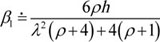(30)

Equations (27) and (28) give the system velocities immediately after impact from rocking about O (realized when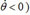. Identical expressions are derived for the case of impact from rocking about O' (realized when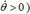.

It is worth noting that the coefficient of restitution e as defined in classical impact theory, relates pre- to post-impact translational velocities normal to the impact surface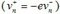, and hence it must not be confused (as often encountered in the literature) with the coefficient of “angular restitution” ε defined in Eq. (29), which relates the pre- to post-impact angular velocities of the body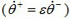. In the derivation presented herein, the coefficient of restitution e enters in the expression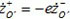which relates pre- to post-impact vertical relative velocities of the impacting corner (O'). The assumption of perfectly inelastic impact is then justified by considering e = 0.

Equation (29) reveals that the coefficient of angular restitution ε depends both on the slenderness ratio λ and the mass ratio ρ. An upper bound for the coefficient of angular restitution is obtained by taking the limit as the slenderness ratio λ approaches infinity: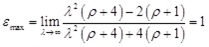(31)

The value ε = 1, implying preservation of the magnitude of the angular velocity after impact, is associated with an energy-lossless impact.

For the assumption of no-bouncing to be satisfied, the coefficient of angular restitution ε should have a positive value. In such a case, the angular velocity of the block will maintain sign upon impact, implying switching pole of rotation from one corner to the other. This requires that: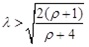(32)

The variation of the coefficient of restitution ε with the slenderness ratio λ is shown in Fig. (3a) for different values of the mass ratio ρ. The strong effect of λ on the coefficient of angular restitution, and hence on the energy dissipated during impact, is evident from this figure. This effect is more pronounced in the lower λ-range (stocky blocks). Similarly, the dependency of coefficient ε on the mass ratio ρ is seen to be weak for very slender blocks, practically diminishing for λ > 8.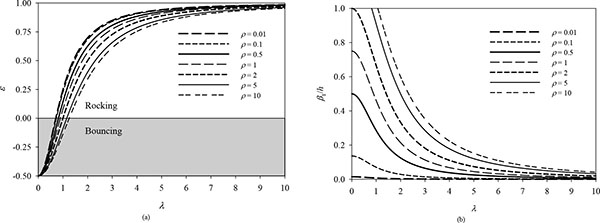Fig. 3. Variation of (a) coefficient of angular restitution ε, and (b) coefficient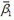with slenderness ratio λ.

The coefficient β1 in Equation (30), which is associated with the reduction of the post-impact linear velocity of the rigid base, depends not only on the parameters λ and ρ, but also on the absolute size of the block (in terms of its height). The normalized coefficient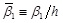is plotted against the slenderness ratio λ for different values of the mass ratio ρ in Fig. (3b). Observe that the value of the coefficient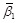decays rapidly with the slenderness ratio λ. As follows from the comparison of Figs. (3a and 3b), the influence of the mass ratio ρ on the coefficientis much greater than that on the coefficient ε.

Equation (28) elucidates the character of base-block dynamic interaction realized upon impact. In effect, the response of the “structure” (rocking block) modifies the input motion of the “foundation” (translating base). This inherent response feature stands in contrast to the dynamic behavior of the Housner-type model, in which the foundation mass is infinite. This interaction ceases to exist when coefficient β1 becomes zero, which by virtue of Eq. (30) occurs when λ → ∞ or ρ → 0. That is to say, the horizontal velocity of base will remain practically unchanged upon impact either in the case of extremely slender block (independently of the block size and value of the mass ratio) or in the case of extremely small block mass relative to the base mass (independently of the block size and slenderness).

#### 2.3.2. Rocking Ceases after Impact

When rocking of the block on top of the translating base ceases, the system will enter the full-contact regime (Fig. (2b)). In this case, the analysis of impact is reduced to calculating the post-impact translational velocity of the system,, given the position and the pre-impact velocities,andBy considering the system as a whole during impact, it can be stated that the horizontal impulse on the system is zero, resulting in the conservation of the system’s linear momentum in the horizontal direction. That is,: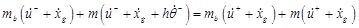(33)

which upon rearranging terms becomes: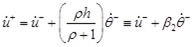(34)

in which coefficient β2 is defined by: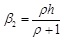(35)

## 3. RESPONSE TO DYNAMIC BASE EXCITATION

The exact equations governing the rocking regime, formulated on the basis of large-displacement approach in Section 2.2, are highly nonlinear and not amenable to exact closed-form solution, even if the time-variation of the excitation is described by a simple analytic function. Accordingly, an ad-hoc computational scheme was developed to calculate the system response under ground excitation. The numerical integration of the equations of motion was pursued in Matlab  through a state-space formulation. The computer program calculates numerically the response of a non-isolated or isolated block subjected to ground excitation under general conditions, considering the different possible oscillation patterns, impact, and arbitrary excitation. In particular, at each time step the program determines the correct oscillation pattern and integrates the corresponding exact nonlinear equations of motion. In addition, close attention is paid to the possibility of transition from one pattern of motion to another and to the accurate evaluation of the initial conditions for the next pattern of oscillation.

An extensive numerical investigation was performed to calculate the dynamic response of the system under simple acceleration pulses and actual pulse-type earthquake records, with the aim of revealing interrelations among the problem parameters and highlighting response trends with respect to the rocking initiation and overturning of the block.

### 3.1. Response to Simple Base-Acceleration Pulses

The response of the system is investigated under simple half- and full-cycle horizontal acceleration pulses. In particular, considered in the analysis are a half-cycle rectangular pulse, a half-cycle sinusoidal pulse, and a full-cycle sinusoidal pulse, characterized by amplitude Ag0 and half-cycle duration td (corresponding to frequency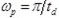), expressed mathematically as follows: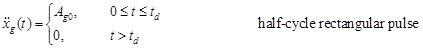(36)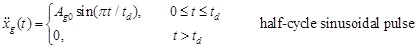(37)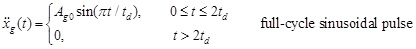(38)

The stability of the isolated block is examined in terms of the minimum amplitude of base acceleration required to overturn the block, by considering the influence of excitation characteristics, the geometric parameters of the block, the inertia parameters of the base/block system, and the constitutive parameters of the isolation system.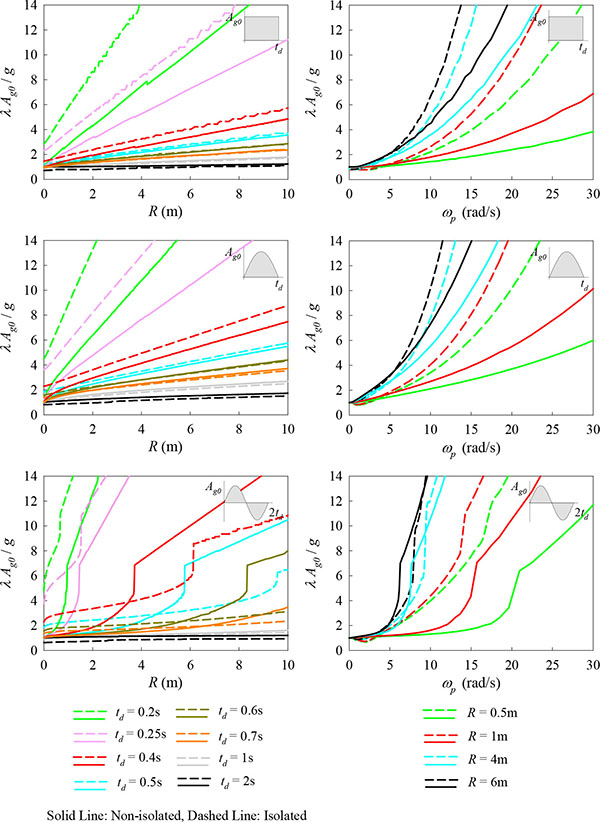Fig. (4). Minimum overturning acceleration as a function of block size (left) and excitation frequency (right) for simple acceleration pulses (λ = 4, ρ = 0.5, Tb = 3.0s, ξb = 0.35).

Fig. (4) plots the normalized minimum overturning base acceleration as a function of block size R for different values of td (left), and as a function of the excitation frequency ωp for different values of R (right) for the simple acceleration pulses used in the analysis. As demonstrated from the left-half of Fig. (4), for the one-sided (rectangular and half-sine) pulses, the isolation has a positive effect on the stability of the block for td < 0.5s or equivalently excitation period Tp < 1s. For the two-sided (full-sine) pulse, this holds true for short-period pulses with td < 0.25s (Tp < 0.5s), while the effectiveness of isolation in the range 0.25<td<0.6s (0.5<Tp<1.2s) is conditional on the size of the block R . That is, the isolation ceases to improve the stability of the block when subjected to such intermediate-period full-sine pulses with increasing block size. Nevertheless, the range of R-values for which the isolation is effective increases as the pulse duration increases. However, it should be noted that, regardless of pulse type, seismic isolation is practically not beneficial (with respect to the overturning potential of the block) for long-period excitations (i.e. Tp > 1s for half-cycle pulses and Tp > 1.2s for full-sine pulses). With reference to the right-half of Fig. (4), the use of isolation results in enhanced behavior as the block size decreases, unless the system is subjected to long-period acceleration pulses.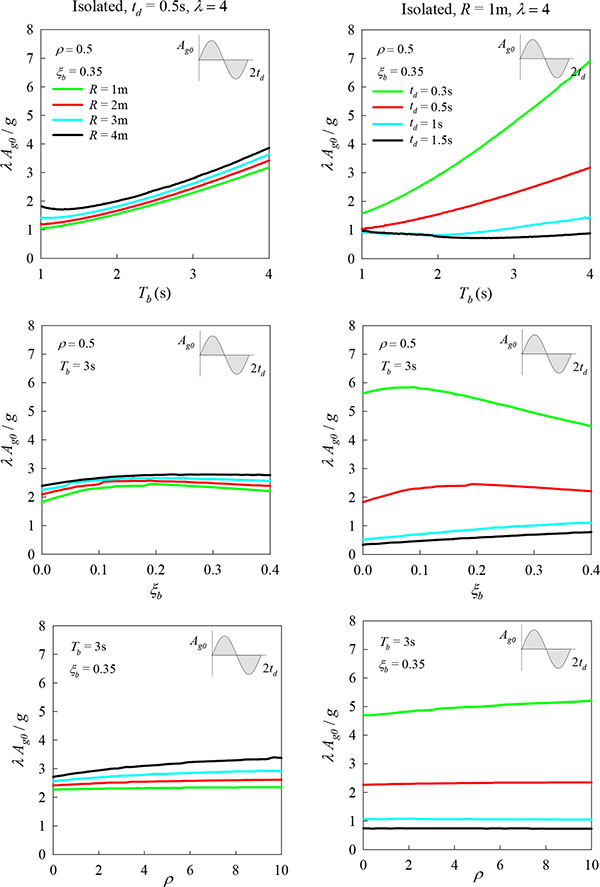Fig. (5). Minimum overturning acceleration as a function of isolation-system parameters Tb, ξb and mass ratio ρ for full-cycle sinusoidal pulse.

Fig. (5) illustrates the influence of isolation-system parameters (Tb, ξb) and mass ratio ρ = m/mb on the stability of the isolated block, for different values of R (left) and for different values of td (right) for full-cycle sinusoidal pulse. As can be seen from Fig. (5), the most influential parameter on the block stability is the isolation-system period Tb. That is, the minimum overturning base acceleration increases with increasing isolation-system period, provided that the pulse duration does not exceed a certain value (roughly 1s). Observe also that the influence of each parameter on the stability is amplified as the pulse duration decreases.

Fig. (6) presents response-regime spectra in the λ-R space for non-isolated and isolated blocks of varying geometric characteristics, for half- and full-cycle pulses with duration td = 0.2s and 0.5s. A linear isolation system is considered in these analyses with Tb = 3s and ξb = 0.35. These spectra depict in a clear way the distinct regimes of block response, with the cyan area indicating “No Uplift”, the green area “Rocking”, and the red area “Overturning” of the block. A total of 6,000 nonlinear dynamic analyses were performed in constructing each behavior map. Each dot in these maps represents the outcome of a single analysis. As illustrated in Fig. (6), the application of seismic isolation yields an increase in the acceleration required for the initiation of rocking. In addition, the spectra plotted in Fig. (6) elucidate a counterintuitive trend observed for bilateral excitations (not observed for unilateral excitations), in terms of the overturning potential of a given input-acceleration amplitude. That is to say, for a given block size R, overturning occurring for certain slenderness λ does not necessarily imply overturning of the block with increasing λ. In mathematical terms, this is equivalent to stating that the (stability) curve defining the boundary between rocking and overturning is not single-valued. By and large, the application of seismic isolation yields a better system performance, with respect to the initiation of rocking and overturning potential of the block, for short-period pulses. On the contrary, for long-period pulses, the use of isolation is not beneficial in improving the stability of the block. Nevertheless, the application of seismic isolation yields an increase in the acceleration required for the initiation of rocking, regardless of the pulse period.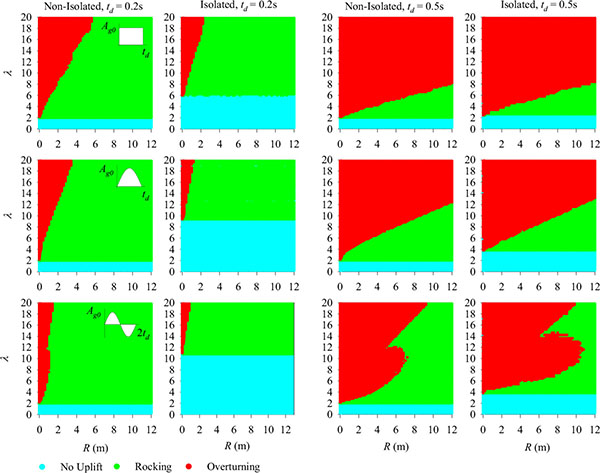Fig. (6). Response-regime spectra in the λ-R space for non-isolated and isolated block of varying geometric characteristics for simple acceleration pulses with td = 0.2s (left) and td = 0.5s (right)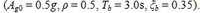.

Fig. (7) compares the response of the block when isolated, considering a linear viscoelastic model with Tb = 3s and ξb = 0.35 and a bilinear hysteretic model (typified by friction-pendulum isolator) with parameters µb = 0.11 and Rb = 2.24m (corresponding to Tb = 3s). In particular, Fig. (7) plots the normalized minimum overturning base acceleration as a function of block size R (left), and as a function of the excitation frequency ωp (right). As can be seen from this figure, the calculated response of the block is comparable for the two isolation-system models. This observation is in agreement with previously published results from analysis using as ground excitation acceleration pulses described by Ricker wavelets . The small discrepancy observed for large R (> 10m), does not affect qualitatively the conclusions drawn above on the basis of a linear isolation model regarding the stability and the rocking incipient condition of the block.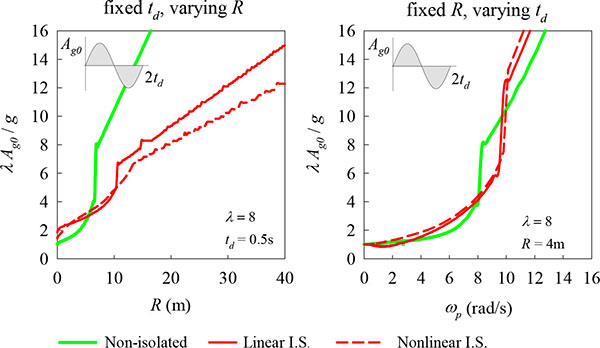Fig. 7. Minimum overturning acceleration for linear and bilinear hysteretic isolation model as a function of block size (left) and excitation frequency (right) for full-cycle sinusoidal pulse.

### 3.2. Response to Earthquake Motions

A wide range of recorded pulse-type ground motions, in terms of amplitude and frequency content, were chosen as input in the analysis of the rocking response of the system. Table 1 lists the characteristics of the motions used for the dynamic analysis.

Table 1. Ground motions used for the dynamic analysis.
Earthquake Station / Component Magnitude(Mw) Distance (km) PGA (g) PGV (m/s) Tp (s)
1966 Parkfield, CA, USA C02/SN 6.20 0.1 0.48 0.75 2.00
1971 San Fernando, CA, USA PCD/SN 6.55 3.0 1.29 1.20 1.47
1978 Tabas, Iran TAB/SP 7.11 1.2 0.85 1.22 5.26
1979 Imperial Valley, CA, USA E04/SN 6.50 6.0 0.36 0.78 4.44
E05/SN 6.50 2.7 0.38 0.92 3.92
E06/SN 6.50 0.3 0.44 1.12 3.85
E07/SN 6.50 1.8 0.46 1.09 3.64
EMO / SN 6.50 1.2 0.38 1.15 2.94
1994 Northridge, CA, USA JFA/SN 6.70 5.2 0.39 1.05 3.03
RRS/SN 6.70 6.0 0.89 1.73 1.25
SCG/SN 6.70 5.1 0.59 1.34 2.94
SCH/SN 6.70 5.0 0.89 1.22 3.03
NWS/SN 6.70 5.3 0.41 1.17 2.70
1995 Aigion, Greece AEG/Long 6.33 6.0 0.50 0.41 0.71
AEG/Tran 6.33 6.0 0.55 0.52 0.68
1999 Izmit, Turkey ARC/SN 7.40 14.0 0.13 0.44 7.14
SKR/SP 7.40 3.1 0.41 0.80 9.52
GBZ/SN 7.40 11.0 0.26 0.41 4.76
GBZ/SP 7.40 11.0 0.03 0.29 6.06
1977 Bucharest, Romania BRI / SN 7.3 190 0.21 0.75 2.13
1994 Northridge, CA, USA Pacoima / PKC090 6.7 8.2 0.30 0.31 0.61
2004 Parkfield Cholame 3W / 360 6.0 8 0.57 0.38 0.52

The stability of the isolated block is again examined in terms of the minimum amplitude of base acceleration required to overturn the block, by considering the influence of excitation characteristics, the geometric parameters of the block, the inertia parameters of the base/block system, and the constitutive parameters of the isolation system.

Representative results are shown in Fig. (8) for the SN-component of the BRI record from the 1977 Bucharest earthquake, and the SN-component of the EMO record from the 1977 Imperial Valley motion (with prevailing period Tp = 2.13s and Tp = 2.94s, respectively, based on Mavroeidis and Papageorgiou, ), as well as the 90-component of the Pacoima Dam record from the 1994 Northridge earthquake and the 360-component of the Cholame-3W record from the 2004 Parkfield event (with prevailing period Tp = 0.61s and Tp = 0.52s, respectively, based on Bray and Rodriguez-Marek, ). In particular, Fig. (8) plots the minimum ground acceleration needed to overturn the block as a function of block size R, for both the non-isolated and isolated case (with isolation-system period Tb= 2s and Tb = 3s). As indicated in this figure, for the short-period Parkfield, Cholame-3W record (with Tp = 0.52s), the isolation system has a positive effect on the stability of the block, provided that the isolation system is designed to have sufficiently large period (case of Tb = 3s). Note that, for the case of the intermediate-period Northridge, Pacoima-Dam record (with Tp = 0.61s), the effectiveness of isolation is conditional on the size of the block R, that is to say the isolation ceases to improve the stability of the block as the block size increases. On the contrary, for the long-period Bucharest, BRI record and Imperial Valley, EMO record (Tp > 2s), the application of seismic isolation does not prove beneficial in improving the stability of the block. It is worthy of noting that these response trends, with respect to the excitation period, are in line with the observed trends for the case of simple full-cycle acceleration pulses.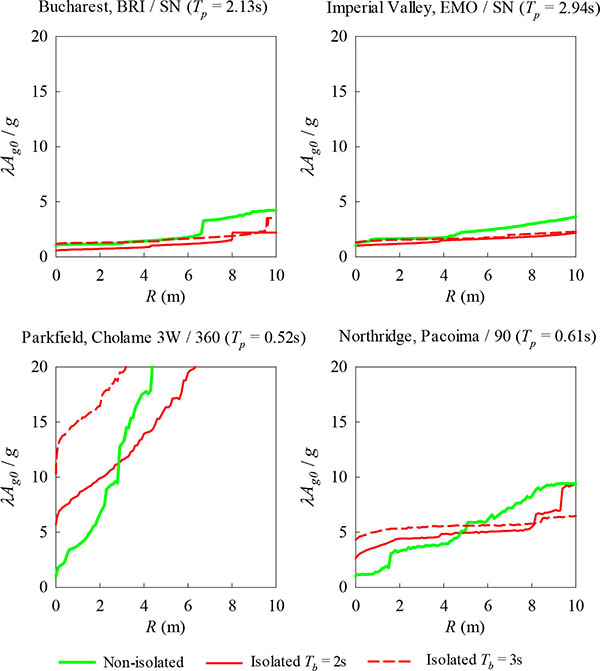Fig. (8). Minimum overturning acceleration for pulse-type earthquake motions with varying frequency content. (λ = 4, ρ = 0.5, ξb = 0.2).

Figs. (9 and 10) depict response-regime spectra in the λ-R space for non-isolated and isolated blocks of varying geometric characteristics, for pulse-type earthquake motions with varying frequency content. These spectra suggest that, regardless of the excitation period, the use of isolation results in an increase in the acceleration required to initiate rocking, a benefit that increases as the isolation period increases. The effectiveness of isolation in increasing the stability of the block is evident in the case of the Parkfield, Cholame-3W record (Tp = 0.52s), the Aigion, AEG/Long record (with Tp = 0.71s), and the Aigion, AEG/Tran record (with Tp = 0.68s). This is also true for the case of the Northridge, Pacoima-Dam record (with Tp = 0.61s), with the exception of very slender blocks (large λ) where the effectiveness of isolation depends on the size of the block R. On the contrary, for the long-period Tabas, TAB record, Imperial Valley, EMO record, the Parkfield, C02 record and the Northridge, NWS record (with Tp > 2s), the presence of isolation is not beneficial with respect to the stability of the block. Similar observations have been made from analysis results with simple full-cycle acceleration pulses.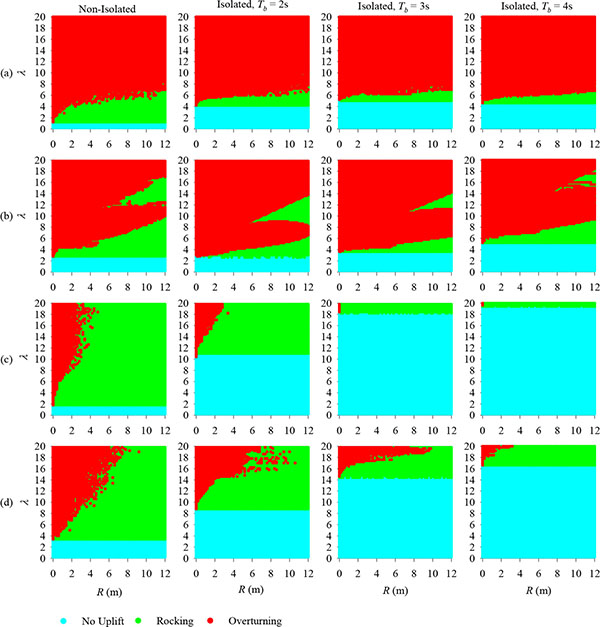Fig. (9). Response-regime spectra in the λ-R space for a non-isolated and isolated block of varying geometric characteristics under (a) Tabas, TAB / SP (Tp = 5.26s), (b) Imperial Valley, EMO / SN (Tp = 2.94s), (c) Parkfield, Cholame 3W / 360 (Tp = 0.52s), and (d) Northridge, Pacoima / 90 (Tp = 0.61s) records. (ρ = 0.5, ξb = 0.35).

The effect of isolation-system parameters on the block behavior is illustrated in Fig. (10) through response-regime spectra in the Tb-ξb space for the considered earthquake records. These spectra specify the values of the constitutive parameters of the isolation system that provide improved performance of the analyzed block subjected to the specific pulse-type base excitations. As seen from Fig. (11), the range of (Tb, ξb) values corresponding to enhanced system performance is considerably larger for the case of short-period records. The effect of block size R is shown in the Tb-ξb spectra of Fig. (12) for the Northridge, Pacoima / 90 record for a given block slenderness λ. Observe that the boundary between no-uplift and rocking regimes (cyan and green areas, respectively) is invariant to the change of block size R, justifying that the initiation of rocking is not dependent on the absolute size of the block (but rather on the height-to-width ratio λ, as Eq. (1) suggests). Moreover, the unfavourable (red) region in the Tb-ξb space entailing overturning of the block is reduced as the block size R increases, a response trend also observed in the case of the simple acceleration pulses (Fig. (5 left)). Evidently, the damping ratio, ξb, has a significant influence on the effectiveness of isolation. In particular, the effectiveness of isolation is reduced as the damping ratio decreases.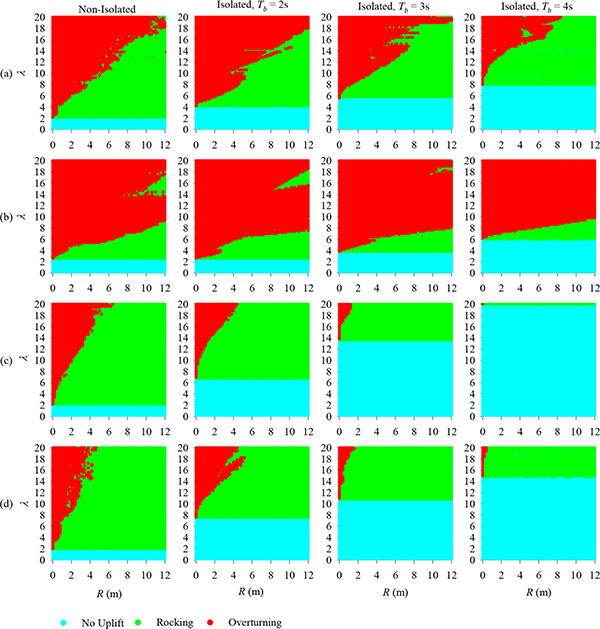Fig. (10). Response-regime spectra in the λ-R space for a non-isolated and isolated block of varying geometric characteristics under (a) Parkfield, C02 / SN (Tp = 2.00s), (b) Northridge, NWS / SN (Tp = 2.70s), (c) Aigion, AEG / Long (Tp = 0.71s), and (d) Aigion, AEG / Tran (Tp = 0.68s) records. (ρ = 0.5, ξb = 0.35).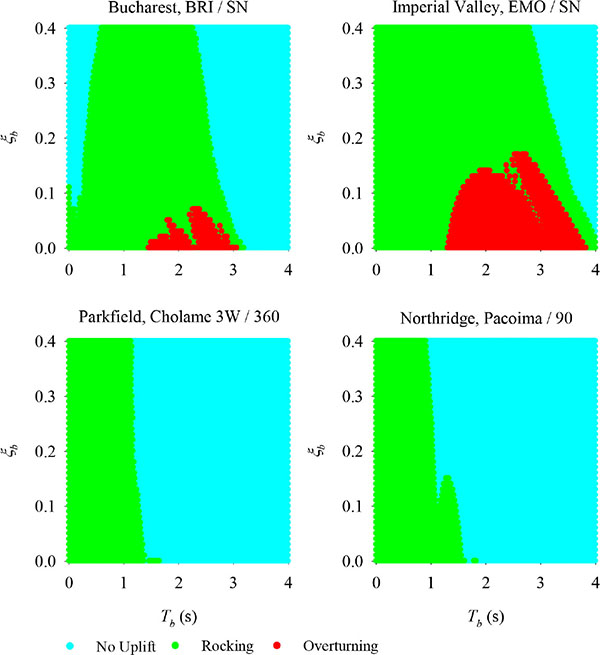Fig. (11). Response-regime spectra in the Tb-ξb space for isolated block under Bucharest, BRI / SN, Imperial Valley, EMO / SN, Parkfield, Cholame 3W / 360 and Northridge, Pacoima / 90 records. (λ = 4, ρ = 0.5, R = 6m).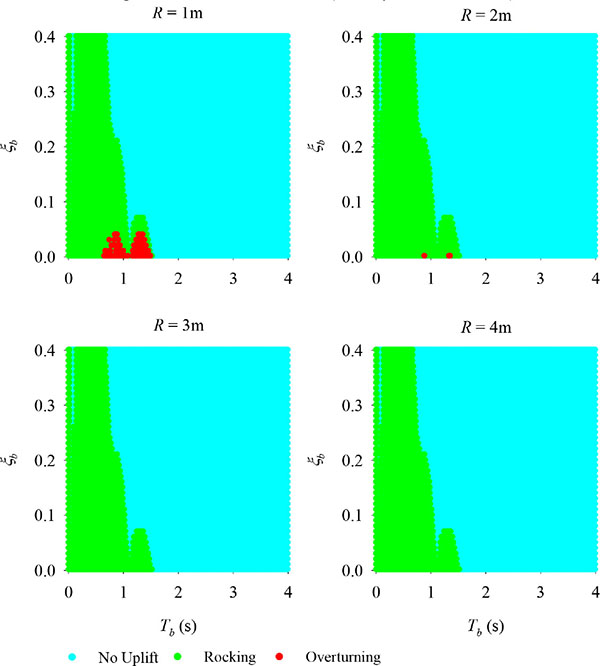Fig. (12). Response-regime spectra in the Tb-ξb space for isolated block of varying size R under Northridge, Pacoima / 90 record. (Tp = 0.61s, λ = 3).

Fig. (13) depicts response-regime spectra in the λ -R space for a wide range of rigid blocks under recorded ground motions using a bilinear hysteretic model with parameters µb = 0.11 and Rb = 2.24s (corresponding to Tb = 3s), and a viscoelastic model with ξb = 0.35 and Tb = 3s. As shown in these figures, the calculated dynamic behavior for the two types of seismic isolation is comparable, with the initiation of rocking (boundary between cyan and green areas) not drastically affected.

## CONCLUSION

A comprehensive mathematical formulation for the nonlinear response of seismically-isolated free-standing rigid blocks to base excitation has been presented. Assuming friction large enough to prevent sliding of the block against the isolation base, when subjected to ground excitation the system can exhibit two possible oscillation patterns, namely “full-contact”, in which the block/base system oscillates as one unit horizontally (1DOF response), and “rocking”, in which the rigid block tilts about its edges as the isolation base translates horizontally (2DOF response). The study has examined the system response through a large-displacement formulation based on the nonlinear equations of motion and an analytical impact model. The mathematical treatment of the problem is broad in scope in that it is neither restricted to small rotations nor slender blocks.

Recognizing the critical role of impact in the dynamics of the system, a rigorous mathematical formulation of the impact problem has been presented in this paper. The model assumes point-impact, perfectly-inelastic impact, and sufficient friction to prevent sliding of the block during impact. Closed-form expressions have been derived for the post-impact velocities of the system in terms of their pre-impact counterparts, thereby defining the coefficient of “angular restitution” ε and the coefficient of “linear restitution” β1 associated with the reduction of the angular velocity of the block and the linear velocity of the supporting base, respectively. The impact model developed elucidates the inherent base-block dynamic interaction, a fundamental response feature that distinguishes the problem at hand from the classic Housner problem of a rocking block impacting a rigid foundation with infinite mass. This interaction ceases to exist (with the velocity of base remaining practically unchanged upon impact) in the case of extremely slender blocks (λ → ∞) or extremely small block-to-base mass ratio (ρ → 0).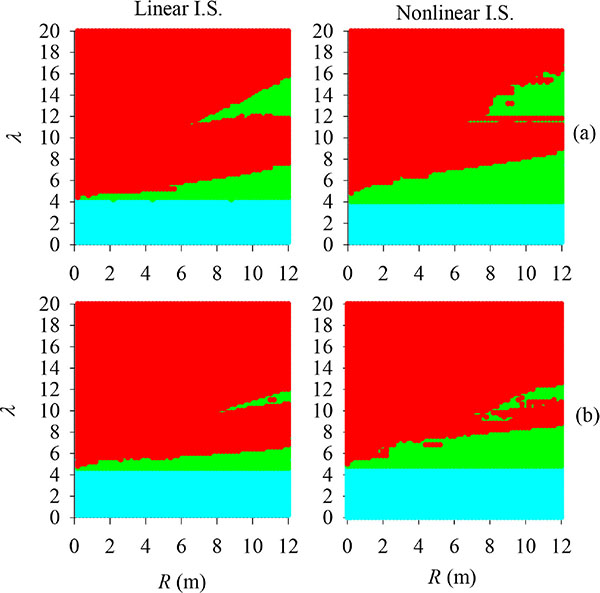Fig. (13). Response-regime spectra in the λ-R space for a class of isolated rigid blocks under (a) the SN component of 1979 Imperial Valley, CA, USA earthquake (EMO station) and (b) the SN component of 1979 Imperial Valley E05 earthquake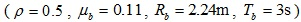.

On the basis of the proposed analytical model, a computer program has been developed to calculate the dynamic response of the system by considering different oscillation patterns, impact occurrence, and arbitrary excitation. An extensive numerical investigation was performed to calculate the dynamic response of the system under simple acceleration pulses and recorded earthquake motions, aiming to identify potential trends in the rocking response and stability of the system. A wide range of recorded pulse-type ground motions, in terms of amplitude and frequency content, were chosen as input in the analysis.

The investigation has shown that, regardless of block size and excitation period, the use of isolation yields an increase in the acceleration required for the initiation of rocking, a benefit that increases as the isolation period increases. This is critical for structures or elements of high importance for which preventing rocking altogether during an earthquake is essential for their effective seismic protection. With respect to the stability of the rocking block, the use of isolation yields a better system performance for smaller-sized blocks both for short- and mid-period excitations, provided that the isolation system is suitably designed. The range of R-values for which the isolation is effective increases with increasing excitation period. On the contrary, for long-period pulses, the application of seismic isolation does not prove beneficial in improving the stability of the block, irrespective of its size. Moreover, the analysis results demonstrated that the choice of isolation-system parameters has a significant influence on the effectiveness of seismic isolation. In particular, the effectiveness of isolation increases as the period of the isolation system Tb increases or as the damping ratio ξb increases. The response of the system has been calculated considering two seismic-isolation models, a linear viscoelastic model and a bilinear hysteretic model. The analysis has demonstrated that the calculated responses on the basis of the two isolation-system models are in good agreement. The study further showed that the response to simple full-cycle acceleration pulses is in line with the observed trends for the case of recorded pulse-type earthquake motions.

### CONFLICT OF INTEREST

The authors confirm that this article content has no conflict of interest.

Declared none.